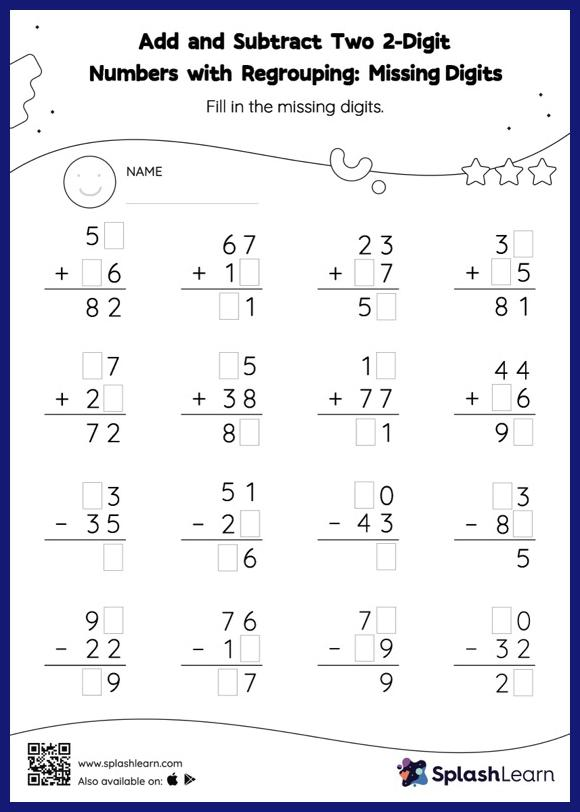# Add and Subtract Two 2-Digit Numbers with Regrouping: Missing Digits Worksheet

Home > Add and Subtract Two 2-Digit Numbers with Regrouping: Missing DigitsTo find the missing number on this worksheet, students can utilize the connection between addition and subtraction. In add and subtract two 2-digit numbers with regrouping worksheet, they must additionally regroup numbers to reach the solution. As the worksheet uses the column method, it is helpful in getting students toward higher accuracy, especially with bigger numbers and in scenarios where regrouping is required.